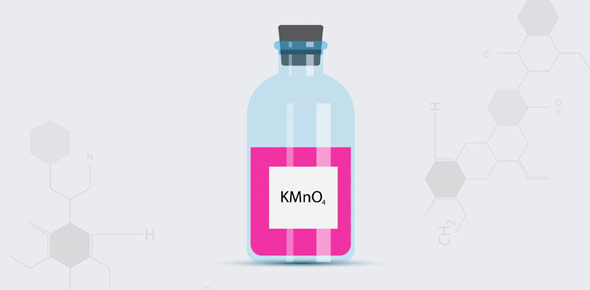# Acid, Bases And Salts

8 Questions | Total Attempts: 839SettingsWhen acids react with bases they usually form salts. How conversant are you with the solutions of this reaction. Take up the simple quiz below and see if indeed you understood the topic on Acids, Bases and Salt. All the best as you tackle it and remember to always revise.

• 1.
Which of the following statement is not true of dilute hydrochloric acid?
• A.

It liberates ammonia gas from ammonium chloride on warming

• B.

It liberates carbon dioxide from carbonates

• C.

It ionises in water to form hydrogen ions

• D.

It reacts with active metals to produce hydrogen

• 2.
Y is heated with aqueous sodium hydroxide and ammonia gas is formed. Y is ________.
• A.

Hydrochloric acid

• B.

Copper (II) oxide

• C.

Ammonium sulphate

• D.

Sodium nitrate

• 3.
Lake Y's water has a pH value of 3. Which substance could be added in to neutralise the pH value of the acidic water present without leaving an alkaline solution.
• A.

Aqueous sodium hydroxide

• B.

Calcium carbonate

• C.

Aqueous ammonia

• D.

Acetic acid

• 4.
Which of the following is true? The strength of an acid depends on:
• A.

It's concentration

• B.

It's pH

• C.

It's solubility in water

• D.

It's degree of ionisation in water

• 5.
Which one of the following burns in air to form an oxide which, when shaken with water, gives a solution with a pH greater than 7?
• A.

Carbon

• B.

Copper

• C.

Nitrogen

• D.

Calcium

• 6.
Which of the following is a soluble base?
• A.

Aluminium oxide

• B.

Copper(II) oxide

• C.

Sodium hydroxide

• D.

Iron(II) hydroxide

• 7.
Which of the following is a reaction observable from this reaction. H2SO4 (aq) + CuCO3 (s) --> H2O (l) + CO2 (g) + CuSO4 (aq)
• A.

An effervescence of colourless gas with a pungent smell is observed

• B.

Copper(II) carbonate reacts with the acid and disappears, forming a blue solution

• C.

Copper(II) carbonate reacts with the acid and disappears, forming a colourless solution

• D.

Copper(II) carbonate reacts with the acid and disappears, forming a pale green solution

• 8.
When two liquids are mixed, a solution with a value of pH 7 is formed. Which of the following are the pH values of the two liquids.
• A.

1st: pH 5, 2nd: pH 2

• B.

1st: pH 5, 2nd: pH 12

• C.

1st: pH 6, 2nd: pH 1

• D.

1st: pH 14, 2nd: pH 7

Related TopicsBack to top